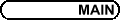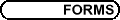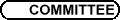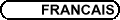Combinatorial and Geometric Group Theory / Théorie des groupes combinatoire et géométrique
Org: Inna Bumagin (Carleton) and/et Dani Wise (McGill)

GREGORY BELL, Penn State University, University Park, PA 16802, USA
Growth of asymptotic dimension of groups

For a metric space X we consider an asymptotic dimension function adX (l) which gives the dimension of X at the "l-scale". As l®¥ this function gives the asymptotic dimension (asdim) of X. The growth of this function is a quasi-isometry invariant, and so its growth is well-defined for finitely generated groups. Examining the growth of adG (l) allows us to consider a notion of dimension for groups with infinite asymptotic dimension. In this paper we examine the growth of adG (l) for groups acting on finite dimensional metric spaces. As a consequence we obtain results for amalgamated products and HNN extensions, among others.

BOB BURNS, York
Research of Yuri Medvedev at York, 1995-1999

Results of Yuri Medvedev from 1996-1999, all involving applications of Lie rings to group theory, will be surveyed.

RUTH CHARNEY, Brandeis University, Waltham, MA 02454, USA
Automorphism groups of spherical and affine type Artin Groups

We use results of Ivanov and Korkmaz on mapping class groups of surfaces to determine the automorphism groups and abstract commensurators of several of the finite and affine type Artin groups.

Joint work with John Crip.

INDIRA CHATTERJEE, Cornell University
Discrete groups, operator norms and distortion

We shall discuss subgroup distortion, and explain how certain geometric properties of a Cayley graph can give an obstruction to having exponentially distorted subgroups.

SEAN CLEARY, The City College of New York
A finitely presented group with unbounded dead-end depth

An element g in a group G is a dead end with respect to a generating set X if it is not adjacent to longer words in the Cayley graph of G with respect to X. It is a "dead end" in the sense that a geodesic ray from the identity to g cannot be extended beyond g. Dead end elements come in different levels of severity, measured by "dead-end depth". In hyperbolic groups, there is always a global bound on the depth of dead-end elements, and Cleary and Taback showed that the dead-end depth of lamplighter groups is unbounded with respect to standard generating sets. The lamplighter examples are not finitely presentable. Here, we find a finitely presentable group where there is no upper bound on the dead-end depth of elements, modelled on Baumslag's construction of a finitely presented metabelian group containing Z wreath Z.

This is joint work with Tim Riley.

ROSS GEOGHEGAN, SUNY at Binghamton
Cocompact proper \operatornameCAT(0) spaces

This is joint work with Pedro Ontaneda. We prove that every cocompact proper CAT(0) space is "almost geodesically complete" aka "almost extendible". A basic ingredient of the proof of this geometric statement is the topological theorem that there is a top dimension d in which the compactly supported integral cohomology of X is non-zero. We also prove that the boundary-at-infinity (with cone topology) has Lebesgue covering dimension d-1. In all this we do not assume that there is any discrete cocompact group of isometries, not even a subgroup having discrete orbits; however, a corollary for the discrete case case is that "the dimension of the boundary" is a quasi-isometry invariant of CAT(0) groups. (By contrast, it is known that the topological type of the boundary is not unique for a CAT(0) group.)

YAIR GLASNER, UIC 851 S Morgan St., Chicago, IL 60607
Primitive groups, in various geometric settings

An action G×X ® X of a group on a set is called primitive if there are no non-trivial G equivariant equivalent relations on X. We say that a group G is primitive if it admits some faithful primitive action on a set. Primitive group actions can be thought of as the "irreducible objects" in the theory of permutation groups.

In the spirit of the well-known theorem of Margulis and Soifer, we classify the finitely generated primitive groups in various geometric settings. Including linear groups, hyperbolic groups, mapping class groups and solvable groups.

In my talk I will describe the results and some applications. Time permitting I will talk about some of the ideas that go into the proof.

This is a joint work with Tsachik Gelander.

BORIS GOLDFARB, University at Albany, SUNY, Albany, NY 12222
On the algebraic structure of geometric group rings

I will show how to use noncommutative localization to relate the question of existence of zero divisors in group rings to existence of certain K-theoretic invariants which were the object of intense study in recent years.

JOINT PANEL DISCUSSION
Participating Sessions

This is a joint Panel Discussion of the following sessions:

• Combinatorial and Geometric Group Theory

• Groups, Equations, Non-commutative Algebraic Geometry

• Interactions between Algebra and Computer Science

JOHN MEIER, Lafayette College
Euler characteristics of automorphism groups of free products

One of the basic questions in group cohomology is determining the Euler characteristic of a group or interesting family of groups. We find and exploit a combinatorial summation identity over the lattice of labelled hypertrees that allows us to gain concrete information on the Euler characteristics of various automorphism groups of free products of groups. In particular, we establish formulae for the Euler characteristics of: the group of Whitehead automorphisms Wh (*i=1n Gi) when the Gi are of finite homological type; Aut(*i=1n Gi) and Out(*i=1n Gi) when the Gi are finite; and the palindromic automorphism groups of finite rank free groups.

This is joint work with Craig Jensen and Jon McCammond.

LUIS RIBES, Carleton University, Ottawa, ON K1S 5B6
Pro-p groups acting on profinite trees

This work describes the structure of countably generated pro-p groups that act continuously on profinite trees under certain conditions. As a consequence one obtains Kurosh-type results describing closed pro-p subgroups of free products of profinite groups, recovering in a unified manner results of Melnikov, Haran, and Ribes-Herfort.

TIM RILEY, Yale
Diameter and filling length of van Kampen diagrams

I will explain recent work with Martin Bridson on the diameter and filling length of van Kampen diagrams and the corresponding filling functions for finitely presented groups.

To measure the diameter of a van Kampen diagram D one uses the path metric on the 1-skeleton of either D itself or the Cayley 2-complex it maps into. We give groups in which there are qualitative differences between the two resulting diameter filling functions.

Filling length controls the length of the contracting loop in the course of a null-homotopy across a van Kampen diagram. We exhibit a group in which the filling length of loops varies dramatically depending on whether or not one keeps a base vertex fixed during the null-homotopy.

YVES STALDER, Université de Neuchâtel, Institut de mathématiques, Rue Emile-Argand 11, CH-2007 Neuchâtel, Suisse
Convergence of Baumslag-Solitar groups

The set of marked groups on two generators can be endowed with a natural topology, so that it becomes a compact Hausdorff totally disconnected space. This framework allows, for instance, a topological definition of Sela's limit groups.

Baumslag-Solitar groups

 BS(m,n) = áa,b | abm a-1 = bn ñ
are canonically marked by the generators a,b. We are interested by the limits of these groups. We will prove that limm,n ® ¥BS(m,n) is the free group on two generators and give some conditions on the sequence (kn)n such that limn ® ¥BS(m,kn) exists. These conditions are related to p-adic integers.

BENJAMIN STEINBERG, Carleton University, 1125 Colonel By Drive, Ottawa ON K1S 5B6
On the spectra of lamplighter-types groups and Cayley Machines

Grigorchuk and Zuk calculated the spectrum and spectral measure of the Markov operator associated to a simple random walk on the lamplighter group Z/2Z\wr Z by realizing it as the group generated by a two-state automaton. In particular, the spectral measure was discrete-a new phenomenon for such Markov operators. Dicks and Schick generalized their results to other wreath product groups G\wr Z, with G a finite group, using a different technique.

If G is a finite group, then the Cayley graph of G (with respect to all generators) can be turned into a Mealy automaton, called the Cayley Machine of G, generating in this way an automaton group. If G is Abelian, one obtains the lamplighter-type group G\wrZ, generalizing Grigorchuk and Zuk who studied the case G = Z/2Z. In general, the group of a Cayley machine is locally finite by infinite cyclic, but is not isomorphic to a wreath product of a finite group with a torsion group. The semigroup generated by the states is free on |G| generators.

Using the techniques of Grigorchuk and Zuk, we compute the so-called Kesten-von Neumann-Serre spectral measures associated to the Schreier graphs with respect to a parabolic subgroups of our groups and the Ihara Zeta function of the infinite Schreier graphs. In the case of G Abelian, we prove the Kesten-von Neumann-Serre spectral measure is the spectral measure associated to the random walk on G\wrZ, recovering the results of Dicks and Schick.

JENNIFER TABACK, Bowdoin College, Brunswick, ME 04011
Geodesic languages for Lamplighter groups and Thompson's group F

We show that both the lamplighter groups in the standard wreath product presentation, and Thompson's group F in the standard finite presentation have infinitely many cone types, and thus no regular language of geodesics. We prove a general theorem about certain types of elements which prohibit a group from having a regular language of geodesics.

Additionally, I will show that each lamplighter group has a context free language of geodesics with unique representatives for each element, and a counter language with the same property.

This is joint work with Sean Cleary and Murray Elder.

FRANCIS TANG, University of Waterloo, Waterloo, ON
Conjugacy separability of Seifert 3-manifold groups over non-orientable surfaces

Seifert 3-manifold groups have nice separability properties. Niblo showed that they are double coset separable. Allenby, Kim and Tang showed that most of the Seifert 3-manifold groups over over orientable surfaces are conjugacy separable. In this talk we discuss the conjugacy separability of Seifert 3-manifold groups over non-orientable surfaces. It turns out all Seifert 3-manifold groups over non-orientable surfaces are conjugacy separable. We conjecture that all Seifert 3-manifold groups are conjugacy separable.

TED TURNER, University at Albany
Test ranks of groups and the Magnus problem FP15

In the Magnus problem list, problem FP15 is the following.

"Let G be an n-generator group. Call a set of elements {g1,...,gk}, a test set for the group G if, whenever

 f(gi) = gi,     i = 1,...,k
for some endomorphism f of G, this f is actually an automorphism of G. The test rank of G is the minimal cardinality of a test set. Can the test rank be equal to 2 if n > 2?" (Posed by Ben Fine.)

I will describe a positive solution. In particular, I will describe how to compute the test rank of any finitely generated abelian group and show that all possible combinations of rank and test rank are realized.

This is joint work with Charles F. Rocca Jr.

KAREN VOGTMANN, Cornell University
Cycles at infinity in Outer space

Using a variation of Bestvina and Feighn's bordification of Outer space, we produce a chain complex which computes the rational cohomology of the group Out(Fn) of outer automorphisms of a free group. We show how to produce a great many cycles in this chain complex, including cycles which give non-trivial cohomology classes in H4 ( Out(F4);Q ) and H8 ( Out(F6);Q ). We conjecture that these cycles span all of the rational cohomology of Out(Fn). The construction of these cycles was inspired by a graphical interpretation of certain cycles, constructed by S. Morita, in a different chain complex which also computes the cohomology of Out(Fn).

This is joint work with Jim Conant.

KEVIN WORTMAN, Cornell University, Department of Mathematics, Ithaca, NY 14853, USA
Finiteness properties of arithmetic groups over function fields

In this talk we'll focus on a proof of Nagao's theorem that SL2(Fq[t]) is not finitely generated. Here Fq [t] is a ring of polynomials with one variable and coefficients in a finite field.

The proof presented will not be new, but I'll explain how it arises as a special case of a new proof used by Kai-Uwe Bux and myself to verify the conjecture that an arithmetic subgroup of a reductive group G defined over a global function field K is of type FP¥ if and only if the semisimple K-rank of G equals 0.

This conjecture had its roots in the work of Serre and Stuhler. Our proof is motivated by the Epstein-Thurston proof that SLn(Z) is not combable when n ³ 3.